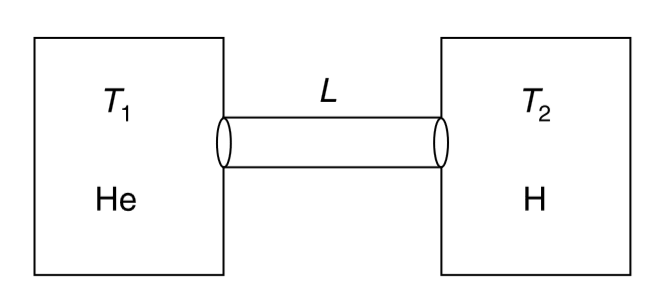# Two identical adiabatic containers of negligible heat capacity are connected by conducting rod of length L and cross sectional area A. Thermal conduct

51 views
in Physics
Two identical adiabatic containers of negligible heat capacity are connected by conducting rod of length L and cross sectional area A. Thermal conductivity of the rod is k and its curved cylindrical surface is well insulated from the surrounding. Heat capacity of the rod is also negligible. One container is filled with n moles of helium at temperature T_(1) and the other one is filled with equal number of moles of hydrogen at temperature T_(2) (lt T_(1)). Calculate the time after which the temperature difference between two gases will becomes half the initial difference.by (90.1k points)
Correct Answer - (15nRL)/(16kA)ln2